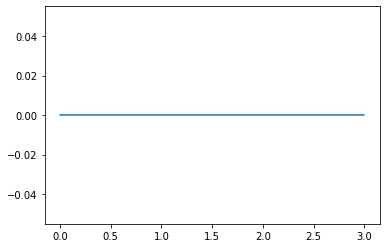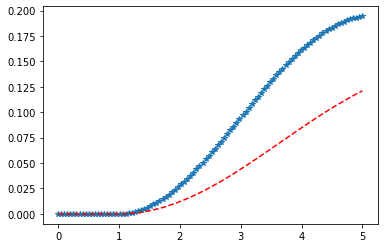Related Articles

# Python – Maxwell Distribution in Statistics

• Last Updated : 31 Dec, 2019

scipy.stats.maxwell() is a Maxwell (Pareto of the second kind) continuous random variable. It is inherited from the of generic methods as an instance of the rv_continuous class. It completes the methods with details specific for this particular distribution.

Parameters :

q : lower and upper tail probability
x : quantiles
loc : [optional]location parameter. Default = 0
scale : [optional]scale parameter. Default = 1
size : [tuple of ints, optional] shape or random variates.
moments : [optional] composed of letters [‘mvsk’]; ‘m’ = mean, ‘v’ = variance, ‘s’ = Fisher’s skew and ‘k’ = Fisher’s kurtosis. (default = ‘mv’).

Results : Maxwell continuous random variable

Code #1 : Creating Maxwell continuous random variable

 `# importing library`` ` `from` `scipy.stats ``import` `maxwell  ``   ` `numargs ``=` `maxwell.numargs ``a, b ``=` `4.32``, ``3.18``rv ``=` `maxwell(a, b) ``   ` `print` `(``"RV : \n"``, rv)  `

Output :

```RV :
scipy.stats._distn_infrastructure.rv_frozen object at 0x000002A9D66DEC88
```

Code #2 : Maxwell continuous variates and probability distribution

 `import` `numpy as np ``quantile ``=` `np.arange (``0.01``, ``1``, ``0.1``) `` ` `# Random Variates ``R ``=` `maxwell.rvs(a, b) ``print` `(``"Random Variates : \n"``, R) `` ` `# PDF ``R ``=` `maxwell.pdf(a, b, quantile) ``print` `(``"\nProbability Distribution : \n"``, R) `

Output :

```Random Variates :
8.999401872992793

Probability Distribution :
[0.00000000e+00 3.70579394e-21 4.46576264e-05 4.02803131e-02
3.15216150e-01 6.42768234e-01 7.96800760e-01 7.98281605e-01
7.24720266e-01 6.27826999e-01]

```

Code #3 : Graphical Representation.

 `import` `numpy as np ``import` `matplotlib.pyplot as plt ``    ` `distribution ``=` `np.linspace(``0``, np.minimum(rv.dist.b, ``3``)) ``print``(``"Distribution : \n"``, distribution) ``    ` `plot ``=` `plt.plot(distribution, rv.pdf(distribution)) `

Output :

```Distribution :
[0.         0.06122449 0.12244898 0.18367347 0.24489796 0.30612245
0.36734694 0.42857143 0.48979592 0.55102041 0.6122449  0.67346939
0.73469388 0.79591837 0.85714286 0.91836735 0.97959184 1.04081633
1.10204082 1.16326531 1.2244898  1.28571429 1.34693878 1.40816327
1.46938776 1.53061224 1.59183673 1.65306122 1.71428571 1.7755102
1.83673469 1.89795918 1.95918367 2.02040816 2.08163265 2.14285714
2.20408163 2.26530612 2.32653061 2.3877551  2.44897959 2.51020408
2.57142857 2.63265306 2.69387755 2.75510204 2.81632653 2.87755102
2.93877551 3.        ]
```Code #4 : Varying Positional Arguments

 `import` `matplotlib.pyplot as plt ``import` `numpy as np ``    ` `x ``=` `np.linspace(``0``, ``5``, ``100``) ``    ` `# Varying positional arguments ``y1 ``=` `maxwell .pdf(x, ``1``, ``3``) ``y2 ``=` `maxwell .pdf(x, ``1``, ``4``) ``plt.plot(x, y1, ``"*"``, x, y2, ``"r--"``) `

Output :Attention geek! Strengthen your foundations with the Python Programming Foundation Course and learn the basics.

To begin with, your interview preparations Enhance your Data Structures concepts with the Python DS Course. And to begin with your Machine Learning Journey, join the Machine Learning – Basic Level Course

My Personal Notes arrow_drop_up